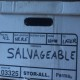Does the Bible make a mistake in claiming that pi equals 3?

It has been alleged that the Bible is in error because it teaches that pi is equal to 3. Recall that pi is the ratio of circumference to diameter in a circle. And even most young students know that pi is not exactly equal to three. It is often approximated as 3.14, though the actual decimal expansion goes on forever: 3.141592653589793. . . . It is not difficult to measure the diameter and circumference of a circle to confirm that pi does indeed have this value. So is the Bible in error?

The relevant passage is 1 Kings 7:23, which states:

Now he made the sea of cast metal ten cubits from brim to brim, circular in form, and its height was five cubits, and thirty cubits in circumference (NASB).

This verse describes a cylindrical vessel built at the order of Solomon. First of all, notice that this passage does not say “exactly ten cubits” or “exactly thirty cubits.” The numbers have been rounded to the nearest integer (or possibly the nearest multiple of ten). Dividing the circumference (30 cubits) by the diameter (10 cubits), we infer that pi is approximately equal to three. But of course, pi is approximately equal to three, so the passage is quite correct.

At best, critics of the Bible could say that the Bible is imprecise here, but they cannot legitimately say that it is inaccurate or mistaken. Even scientists today will round off numbers at appropriate times. Remember that any decimal expression of pi must be rounded at some point anyway, since the expansion is infinite. There is no fallacy in rounding a number.

Second, we should consider the matter of significant figures. On a physics test, if a circle is said to have a diameter of 10 feet and the student is asked to compute the circumference, the correct answer is 30 feet — not 31 feet. The reason 31 feet is an incorrect answer is because it implies a precision that is unwarranted by the given information. The value 10 feet indicates that the diameter has been rounded. Perhaps it has been rounded up from the exact value of 9.5 feet, in which case the exact diameter would be 29.845 . . . feet — which rounds up to 30 feet.

Third, we should consider 1 Kings 7:26, which states that this cylindrical vessel “was a handbreadth thick.” Since the diameter is given from “brim to brim” (verse 23), the 10 cubits is referring to the outer diameter (which includes the handbreadth thickness of the rim). However, the circumference may well refer to the inner circle (as this is more representative of the pool of water inside the cylinder), which excludes the handbreadth. So even if we take the outer diameter to be exactly 10 cubits, the inner diameter would be smaller. A handbreadth is roughly 1/4 of a cubit; so, the inner diameter would be 10 cubits — (0.25 x 2) cubits = 9.50 cubits. This means the inner circumference would be 29.845 . . . cubits, which rounds up to 30 cubits (not 31 cubits).

In conclusion, the accusation that the Bible has made a mathematical mistake is totally without merit. The biblical answer is spot on, given the information presented and the precision of the numbers in question.

## 2 thoughts on “As Easy as Pi”

1.Salvageable says:
1.curryNcode says: